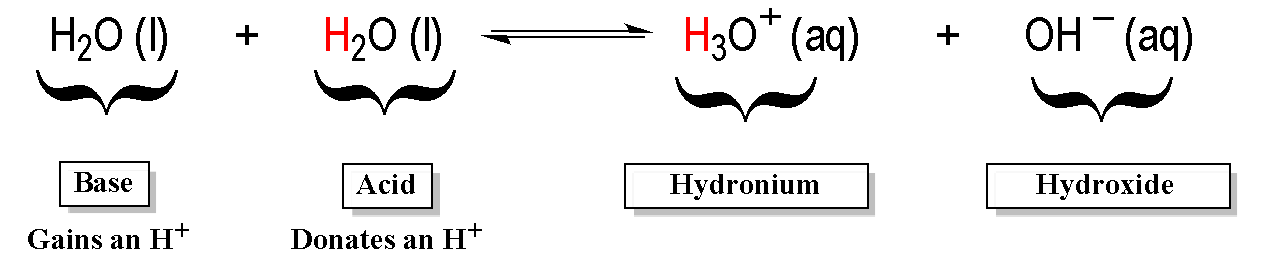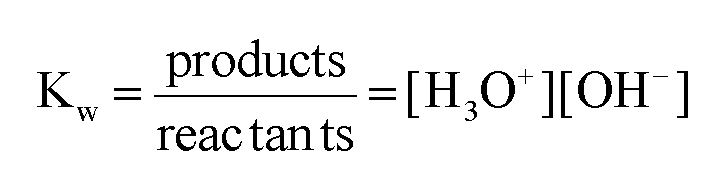Clutch Prep is now a part of Pearson
Ch.10 Acids and BasesWorksheetSee all chapters

# Auto-Ionization

See all sections
Sections
Acid-Base Introduction
Arrhenius Acid and Base
Bronsted Lowry Acid and Base
Acid and Base Strength
Ka and Kb
The pH Scale
Auto-Ionization
pH of Strong Acids & Bases
Acid-Base Equivalents
Acid-Base Reactions
Gas Evolution Equations (Simplified)
Ionic Salts (Simplified)
Buffers
Henderson-Hasselbalch Equation
Strong Acid Strong Base Titrations (Simplified)

In a Self-Ionization reaction two water molecules react with one another, where one acts an acid and the other as a base.

Concept #1: Understanding the Auto-Ionization Reaction.

Transcript

Hey guys, in this new video we are going to take a look at the auto ionization of water. So what we’re going to see here is in an aqueous solution so the solvent is water, water molecules can react with each other. And we’re going to say that this is called a process of auto ionization or self-ionization. Now in this process we’re going to create H3O+ and OH-. I just realized, how does this happen? For this to occur, one of the water molecules has to act as an acid and the other one has to act as a base. So let’s say that this one here acts as the acid, and this here is the base. Remember, Bronsted-Lowry definitions, the acid acts as an H+ donor, the base acts as an H+ acceptor.
So this base right here is going to accept an H+ and as a result it’s going to become H3O+. Then we’re going to say that the other water molecule, the one that’s acting as an acid because it’s giving away an H+ it becomes OH-. And just also realize here that water molecules exist in liquid form but then once they become these ions, the hydronium ion and the hydroxide ion, they’re going to be aqueous. They’re no longer liquid water and because of that they’re no longer a liquid they’re going to be aqueous. Remember aqueous just means that the parts of the water are attracted to these particular ions. We can simply auto ionization or self-ionization by just saying that water separates into H+ plus OH-. remember we said this before that H+ is the same thing as H3O+. Both are hydronium ion, they both mean the same exact thing.
Now we’re going to say here that the equilibrium equation for water is called the ion product. An ion product is simply said to be KW. So we aren’t talking about the KW for water, we’re talking about the ion product of water. Now we’re going to say that KW= your hydronium ion multiplied times your hydroxide ion. And we’re going to say here that at 25 degrees Celsius, KW is 1.0 times 10 to the negative 14. But remember just like all K’s it’s going to be temperature dependent. If you’re going to change the temperature you’re going to change the value of the KW. We’re going to say increasing the temperature will increase our KW.
Now here I give us a bunch of different values for KW found at various temperatures. And notice as the temperature gradually increases our KW will get bigger and bigger. Now just realize on your exam when you need to use KW if they don’t give you a temperature, just assume that it’s 25 degrees Celsius. So then KW would be 1.0 times 10 to the negative 14. Knowing this, let’s take a look at this question here.The ionization product of Kw is used in this self-ionization equation and ignoring solids and liquids gives the equilibrium equation as:Example #1: Determine the concentration of hydronium ions for a neutral solution at 25oC and at 50oC.

Transcript

This question states, determine the concentration of hydronium ion. Basically, find H+ or H3O+. They both mean the same thing. For a neutral solution at 25 degrees Celsius and 50 degrees Celsius.
What we should realize here is what does neutral mean. Neutral means that your compound is not acidic or basic. And what it really means is that H3O+ equals OH-. They’re both the same thing. And because they’re equal to each other, we’re going to set them both to an unknown variable. We’re going to set them to x. Then what we’re going to do here is we know at 25 degrees Celsius KW will be 1.0 times 10 to the negative 14. So at 25 degrees Celsius that’s what our KW is. And we just said that H3O+ and OH- are equal when the solution is neutral and we’re going to set them both equal to x. So then you’re going to say x times x really means x squared. Now you only want to find x, not x squared so take the square root. In this case, x=1.0 times 10 to the negative 7. That number right there represents my concentration of H3O+ and technically because it’s neutral, it also represents the concentration of OH-. If you wanted to do it at 50 degrees Celsius, we’d have to realize that since the temperature changes, KW changes to this new number here. We’re going to say 5.476 times 10 to the negative 14, same exact process, equals x squared. We just want x so we’re going to take the square root of both sides. When we do that, it’s going to give us a final answer of x=2.34 times 10 to the negative seven.
Remember, this is important to know because KW equals H+ and sometimes OH-. This equation is important because if the temperature is not given, assume that KW equals 1.0 times 10 to the negative 14. If you know H+ concentration, then you know OH- concentration because KW will be our constant and vice versa. If you know OH-, you can find H+. This is the relationship we need to understand and these are the beginning steps for us to figure out the pH and pOH of different types of aqueous solutions.